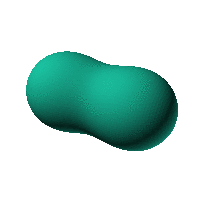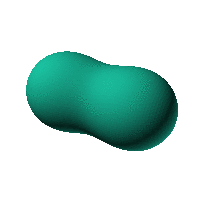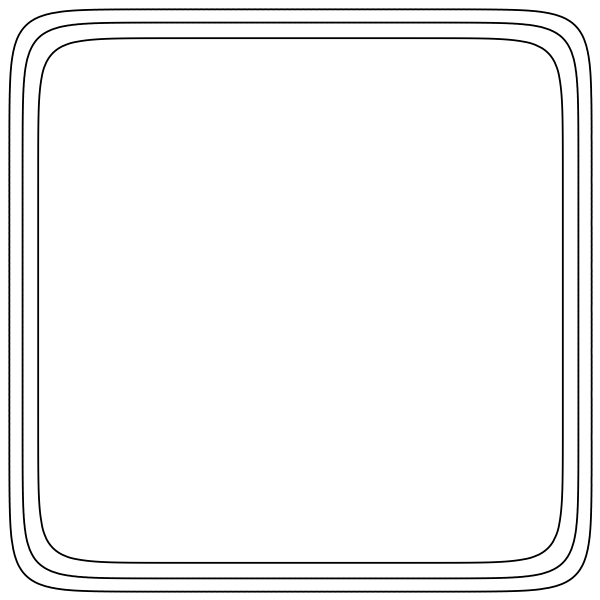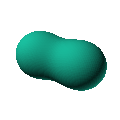Blob Farm# blob53The blob in all its glory:This is derived from two spheres next to each other. Maybe it could be the start of a spaceship or camper.

 Click on the snapshot to download the blob's stl file.Octave Code:
```  # name of the blob
project = "blob53";

# function at origin must be <0, and >0 far enough away.  w=0 defines the surface
function w = f(x2,y2,z2,c,r)  # three lobed toroid with low profile bumps and flat top
x = (x2-c(1))/r(1);
y=(y2-c(2))/r(2);
z=(z2-c(3))/r(3);
w=-44.9375-20.5*x.^2+x.^4+4.5*y.^2+2*x.^2.*y.^2+ ...
y.^4+4.5*z.^2+2*x.^2.*z.^2+2*y.^2.*z.^2+z.^4;
endfunction;

c_outer = [0,0,0];
r_outer = [28,28,28];

step = 4;  # grid pitch in mm  start with 4mm to see the shape quickly.  Once you have it just right, change to 2mm for printing

xmin = -150;
xmax = 150;

source("../octave/func2stl_v01.m");  # do all the calculations
```
GNU Octave